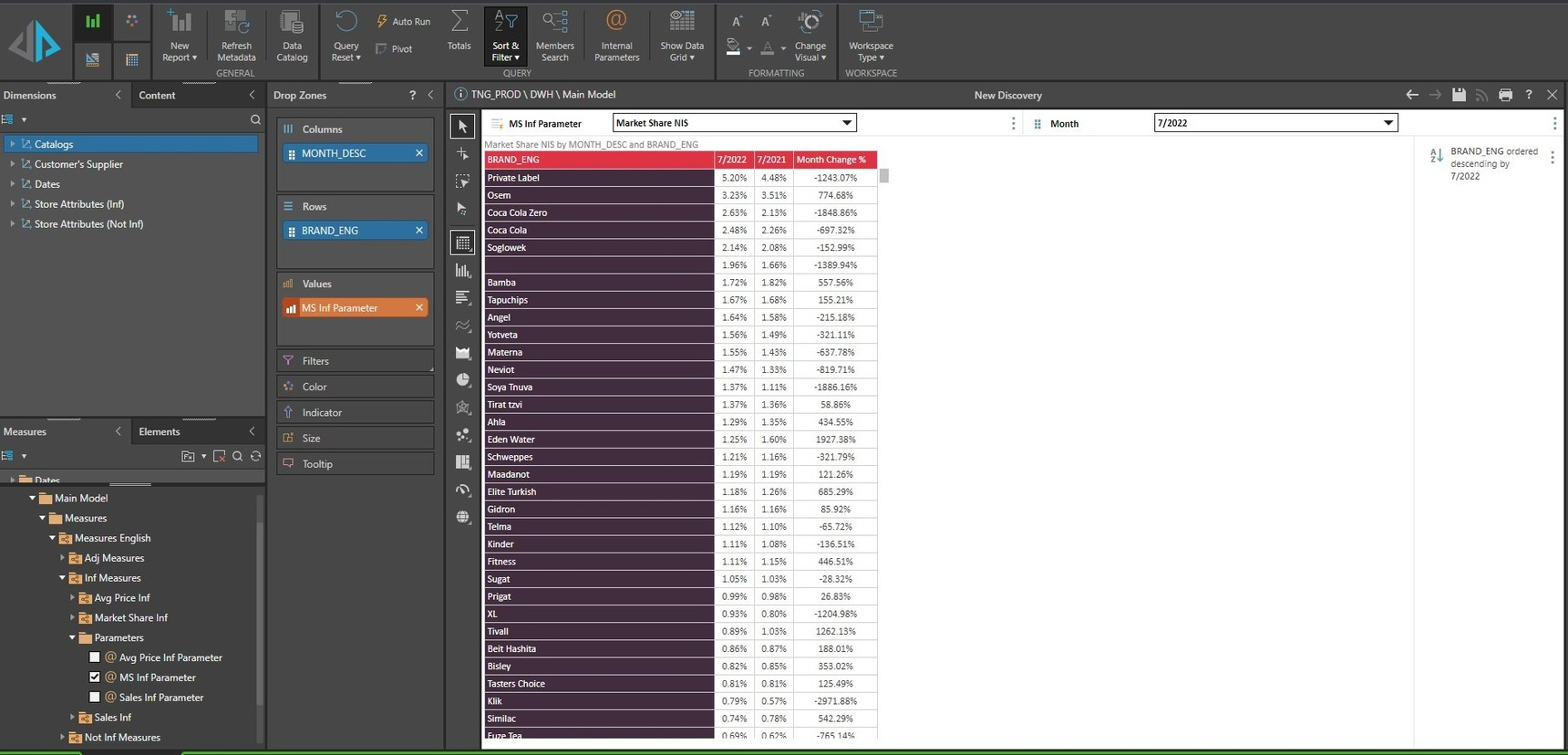0# Percentage points changeHi

We are looking at a solution to create point percentage change column.

In the given example we would like to create a measure that can be calculated on percentages, but will be presented as a number.

For example, in the first line here, we are looking at 5.2% - 4.48%, while a required result would be 0.72 (without the % sign).

Even if the define the measure as a number in formulate, it would still show % in discovery.

Any ideas?

Many thanks

3replies Oldest first
• Oldest first